## Introduction to databases

database: large and persistent collection of factual data and metadata organized to facilitate efficient retrieval and revision

data model: determines the nature of the metadata and how retrieval and revision is expressed

database management system (DBMS): program (or set of programs) that implements a data model

schema: collection of metadata conforming to an underlying data model

database instance: collection of factual data as defined by a given database schema

### Three level schema architecture

external schema (view): what application programs and user see

conceptual schema: description of logical structure of all data in database

physical schema: description of physical aspects (files, devices, storage algorithms, etc)

### Data independence

Idea: Applications do not access data directly but, rather through an abstract data model provided by the DBMS

physical data independence: applications immune to changes in storage structures

logical data independence: modularity

• ex. the WAREHOUSE table is not accessed by payroll app; EMPLOYEE table is not accessed by inventory control app

### Transaction

When multiple applications access the same data, undesirable results occur.

transaction: an application-specified atomic and durable unit of work

ACID properties:

• atomic: a transaction occurs entirely, or not at all
• consistency: each transaction preserves the consistency of the database
• isolated: concurrent transactions do not interfere with each other
• durable: once completed, a transaction’s changes are permanent

### DBMS interface

data definition language (DDL): for specifying schemas

data manipulation language (DML): for specifying retrieval and revision requests

### Summary

DBMS helps:

• to remove common code from applications
• to provide uniform access to data
• to guarantee data integrity
• to manage concurrent access
• to protect against system failure
• to set access policies for data

## The relational model

Set comprehension for questions:

$\{(x_1, ..., x_k) | \langle condition \rangle\}$

Answers: all k-tuples of values that satisfy $$\langle condition \rangle$$

### Relational database components

universe: a set of values $$\textbf{D}$$ with equality ($$=$$) defined

relation: predicate name $$R$$, and arity $$k$$ of $$R$$ (# of columns)

• instance: a relation $$\textbf{R} \subseteq \textbf{D}^k$$

database: signature: finite set $$\rho$$ of predicate names

• instance: a relation $$\textbf{R}_i$$ for each $$R_i$$

signature: $$\rho = (R_1, ..., R_n)$$

instance: $$\textbf{DB} = (\textbf{D}, =, \textbf{R}_1, ..., \textbf{R}_n)$$

### Simple truth

Relationships between objects (tuples) that are present in an instance are true, relationships absent are false

### Conditions in relational calculus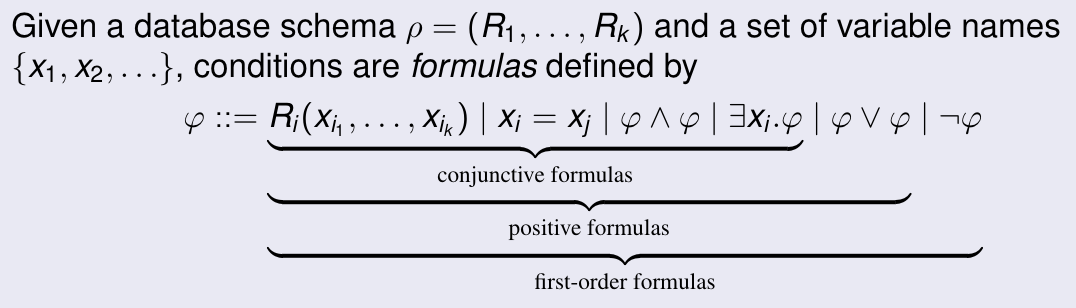### Integrity constraints

yes/no conditions that must be true in every valid database instance

### Domain independent query

domain independent query: a query $$\{(x_1, ..., x_k) \vert \phi\}$$ such that

$\textbf{DB}_1, \theta \models \phi \iff \textbf{DB}_2, \theta \models \phi$

for any pair of database instances and for all $$\theta$$

### Range restricted query

range restricted formula: a formula $$\phi$$ such that for $$\phi_i$$ that are also range restricted, $$\phi$$ has the form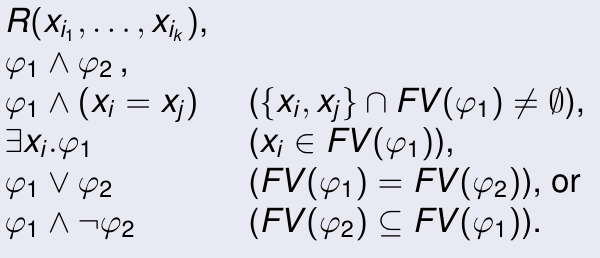Theorem: range restricted $$\implies$$ domain independent

Theorem: every domain independent query can be written equivalently as a range restricted query

### What cannot be expressed in relational calculus?

RC is not turing complete

It cannot express:

1. built in operations (ordering, arithmetic, string operations, etc)
2. counting/aggregation (cardinality of sets)
3. reachability/connectivity (paths in a graph)

TODO

TODO

## Entity relationship model

ER model

World described in terms of

• entities: distinguishable object
• attributes: describe properties of entities or relationships
• relationships: represent certain entities are related to eachother

Examples: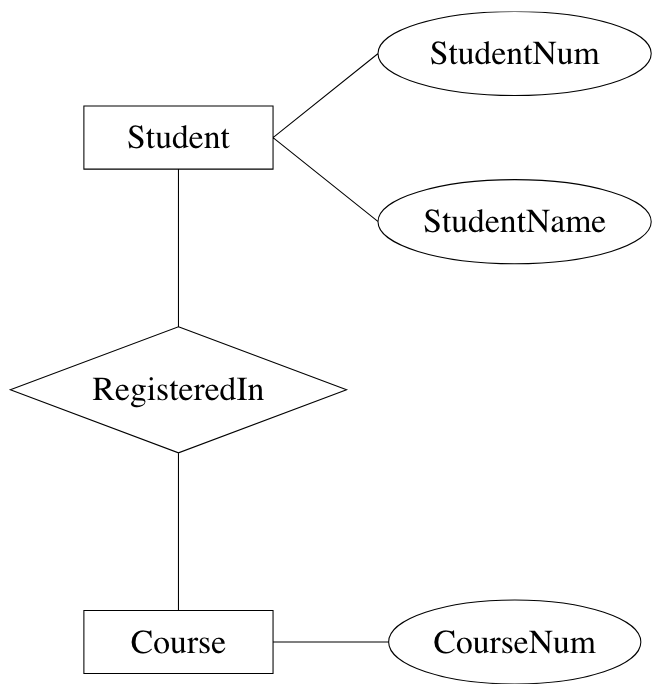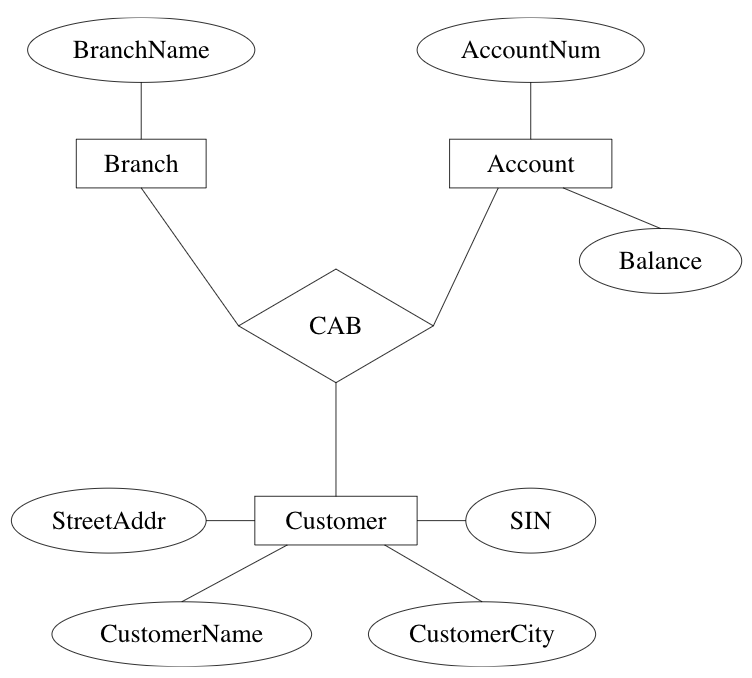role: function of an entity set in a relationship set

• required when entity set has multiple functions in a relationship set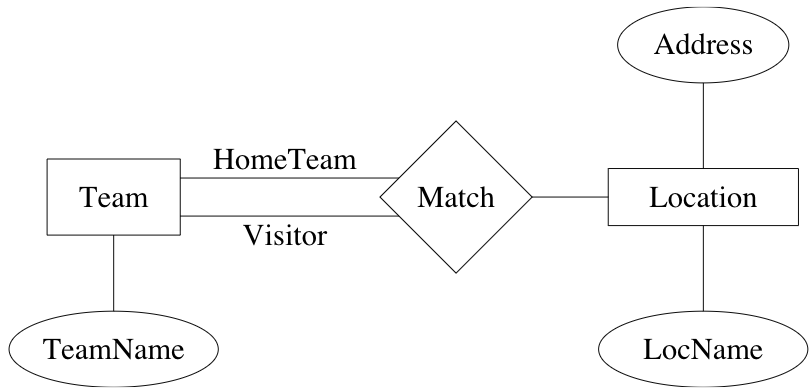primary key: selection of attributes whose values determine a particular entity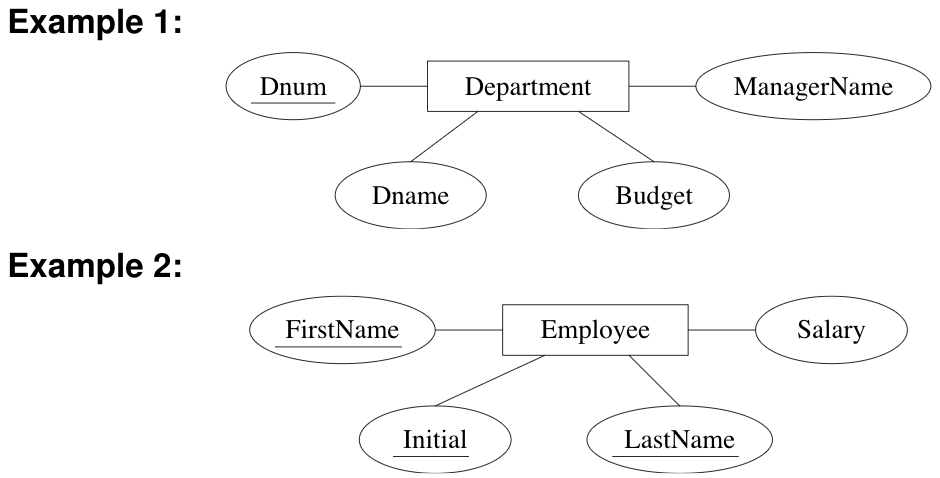many-to-many (n:n): entity in one set related to many entities in other set, and vice versa (default)

many-to-one (n:1), one-to-many (1:n): entity in one set can be related to at most one entity in the other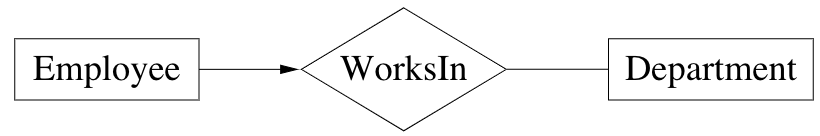one-to-one (1:1): obviousexistence dependency: if x is existence dependent on y, then

• y is a dominant entity
• x is a subordinate entity

weak entity set: entity set containing subordinate entities

• must have a n:1 relationship to a distinct entity set

strong entity set: entity set containing no subordinate entities

Example: transactions are existence dependent on accounts. All transactions for a given account have a unique transaction number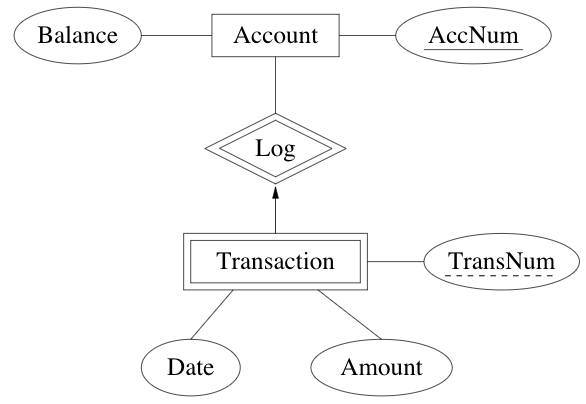discriminator of a weak entity set: set of attributes that distinguish subordinate entities of the set, for a particular dominant entity

• primary key for a weak entity set: discriminator + primary key of entity set for dominant entities

### Extensions to E-R modeling

structured attributes

• composite attributes: composed of fixed # of other attributes
• multi-valued attributes: attributes that are set-valued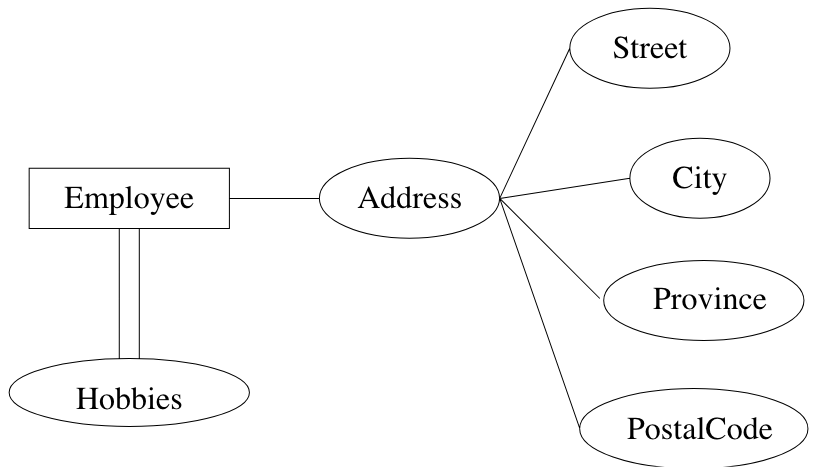aggregation: view relationship as higher level entity

Example: accounts are assigned to a given student enrollment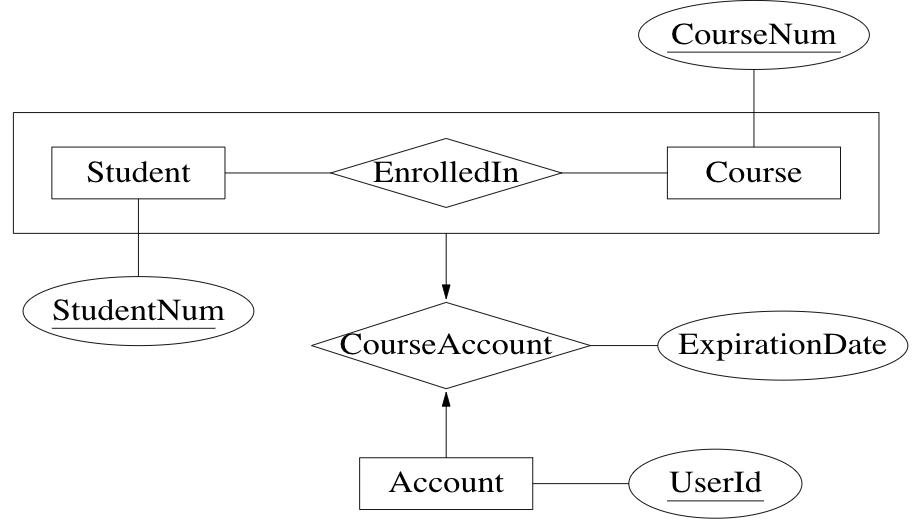specialization: specialized kind of entity set may be derived from a given entity set

Example: grad students are students who have a supervisor and a # of degrees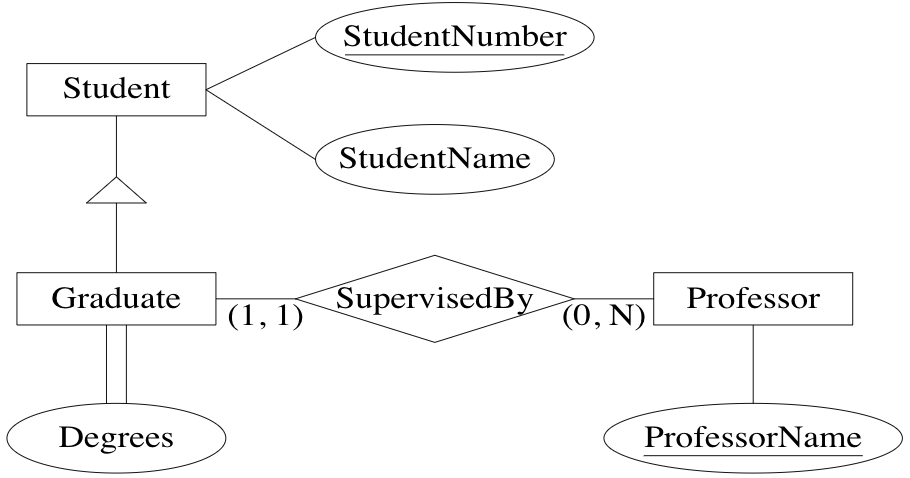generalization: several entity sets can be abstracted by a more general entity set

Example: vehicle abstracts the notion of a car and a truck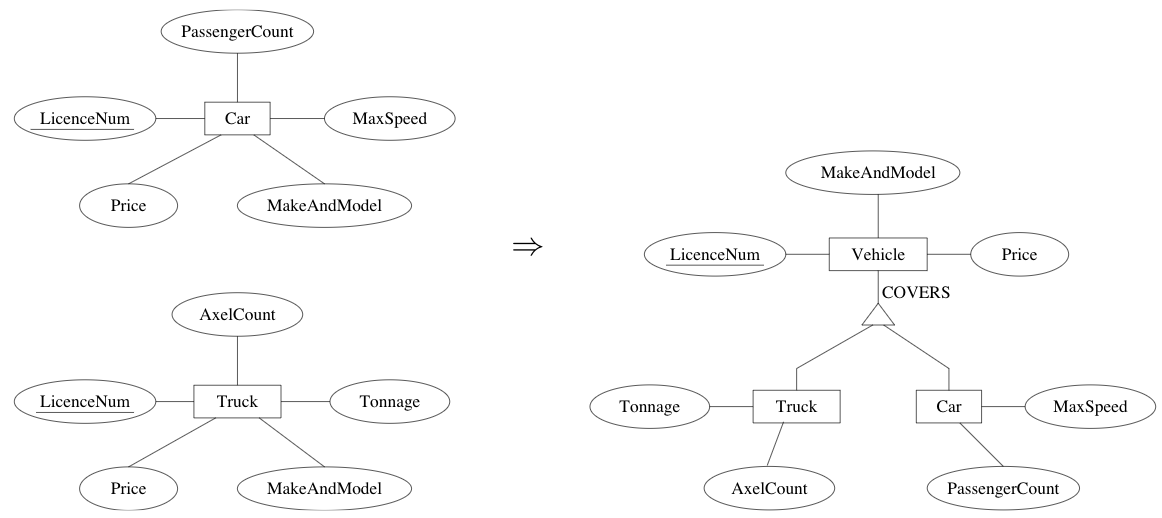disjointness: specialized entity sets are usually disjoint but can be declared to have entities in common

Example: to accommodate utility vehicles, they can be both a car and truck via OVERLAPS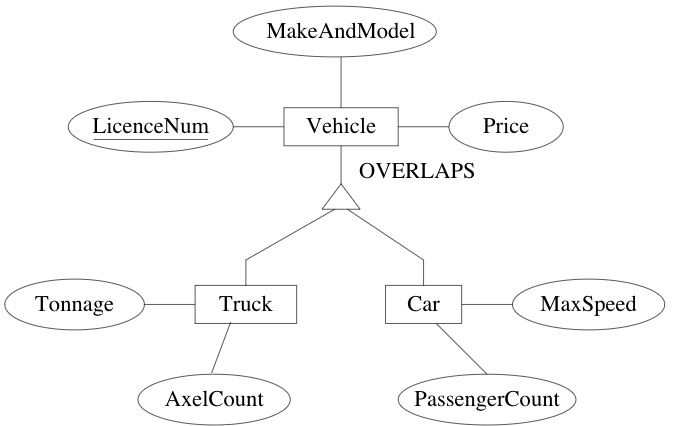### Exampe: registrar database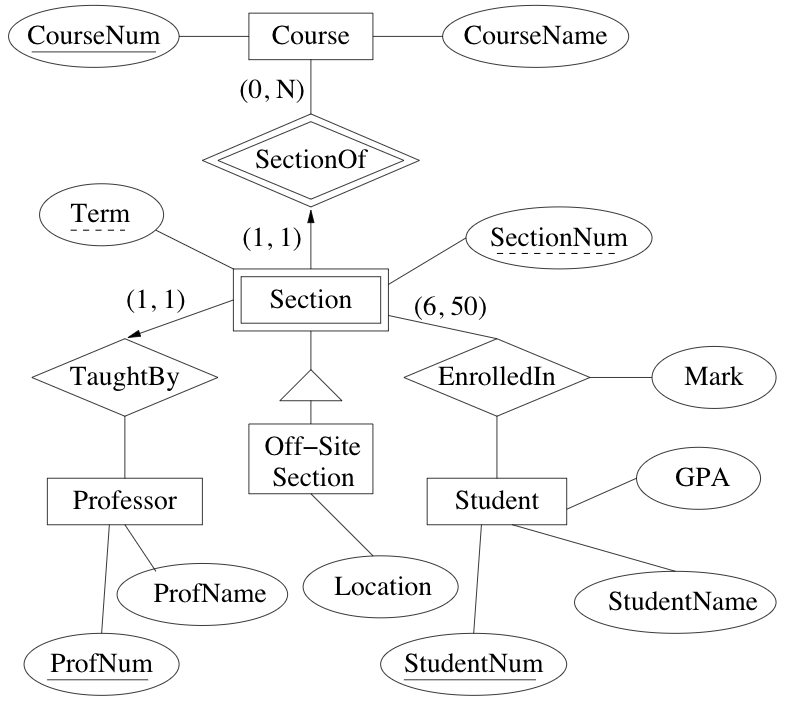## Normal Forms and Dependencies

### Anomalies

Problems that occur in poorly planned, un-normalized databases where all data is stored in one table.

insertion anomaly: impossible to add a piece of data without adding a related piece of data

• ex. library db cannot create a new member until he/she takes out a book

deletion anomaly: a piece of data may be validly deleted, causing the unintentional deletion of another piece of related data

• ex. deleting the last loan of a book may delete all information about the book from the database

### Functional Dependencies

Expresses the fact that in a relational schema, the values of a set of attributes uniquely determines the values of another set of attributes.

Notation: $$X \rightarrow Y$$ means $$X$$ functionally determines $$Y$$

Example: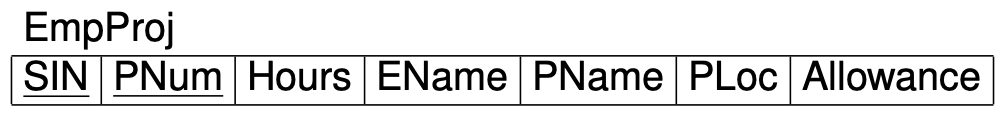• SIN $$\rightarrow$$ EName
• PNum $$\rightarrow$$ PName, PLoc
• PLoc, Hours $$\rightarrow$$ Allowance

#### Implication for FDs

A set $$F$$ logically implies a FD $$X \rightarrow Y$$ if $$X \rightarrow Y$$ holds in all instances of $$R$$ that satisfy $$F$$.

Closure $$F^+$$ of $$F$$ is the set of all FDs that are logically implied by $$F$$.

• then $$F \subseteq F^+$$

Example: If $$F = \{A \rightarrow B, B \rightarrow C\}$$, then $$F^+ = \{A \rightarrow B, B \rightarrow C, A \rightarrow C\}$$

#### Armstrong’s axioms

reflexivity: $$Y \subseteq X \implies X \rightarrow Y$$

augmentation: $$X \rightarrow Y \implies XZ \rightarrow YZ$$

transitivity: $$X \rightarrow Y, Y \rightarrow Z \implies X \rightarrow Z$$

These axioms are

• sound: anything derived from $$F$$ is in $$F^+$$ (ie. anything you can prove is correct)
• complete: anything in $$F^+$$ can be derived (ie. anything that is correct can be proved)

union: $$X \rightarrow Y, X \rightarrow Z \implies X \rightarrow YZ$$

decomposition: $$X \rightarrow YZ \implies X \rightarrow Y$$

Example: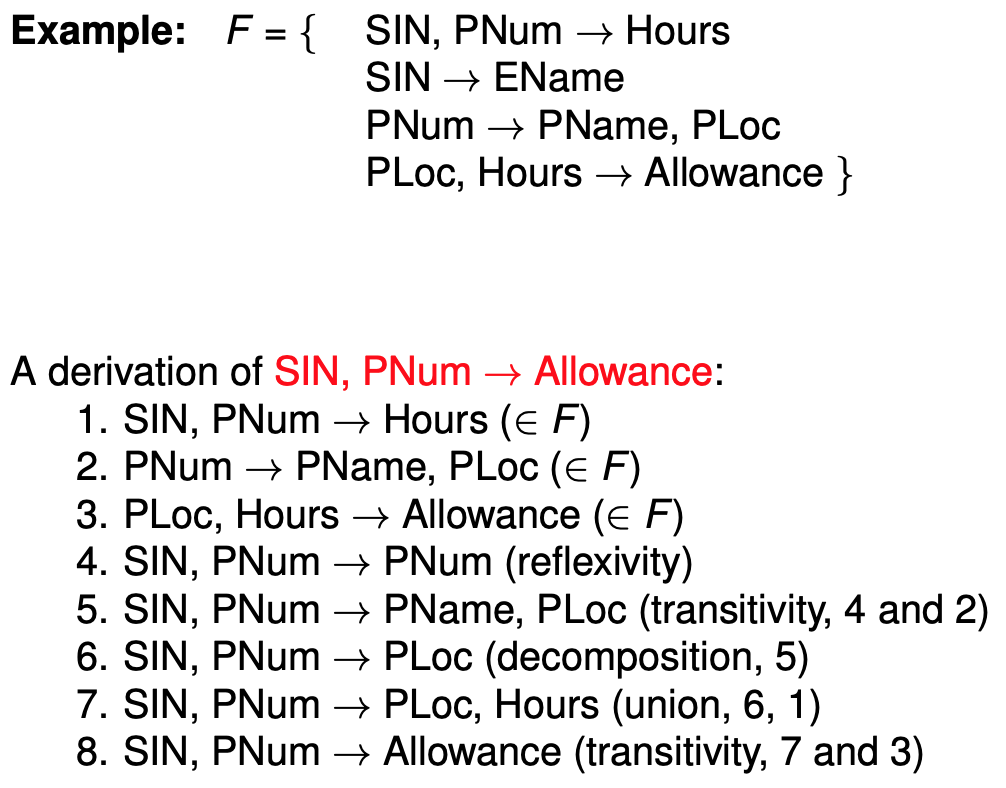### Keys

$$K \subseteq R$$ is a superkey for relation schema $$R$$ if dependency $$K \rightarrow R$$ holds on $$R$$

$$K \subseteq R$$ is a candidate key for relation schema $$R$$ if $$K$$ is a superkey and no subset of $$K$$ is a superkey

primary key: a chosen candidate key

### Decompositions

The collection $$\{R_1,...,R_n\}$$ of relation schemas is a decomposition of relation schema $$R$$ (= set of attribute) if

$R = R_1 \cup ... \cup R_n$

Good decompositions

• do not lose information
• do not complicate checking of constraints
• do not contain anomalies

#### Lossless-Join Decompositions

A decomposition $$\{R_1, R_2\}$$ of $$R$$ is lossless iff the common attributes of $$R_1$$ and $$R_2$$ form a superkey for either schema, that is

$R_1 \cap R_2 \rightarrow R_1 \vee R_1 \cap R_2 \rightarrow R_2$

In other words, re-joining the decomposition always produces the exact same tuples as in the original relation $$R$$.

Example: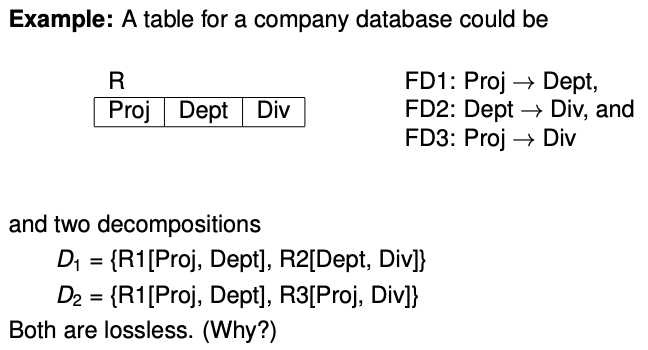• $$R_1 \cap R_2$$ (= Dept) is a superkey of $$R_2$$
• $$R_1 \cap R_2$$ (= Proj) is a superkey of both $$R_1$$ and $$R_3$$
• decomposition $$D_1$$ is better because FD1 and FD2 can be tested on the two separate tables. FD3 then follows from transitivity. FD2 is an interrelational constraint in decomposition $$D_2$$ because testing it requires joining tables $$R_1$$ and $$R_3$$

A decomposition $$D = \{R_1, ..., R_n\}$$ is dependency preserving if there is an equivalent set $$F'$$ of FDs, none of which is interrelational in $$D$$.

### Normal forms

#### Boyce-Codd Normal Form (BCNF)

Schema $$R$$ is in BCNF w.r.t. $$F$$ iff whenever $$X \rightarrow Y \in F^+$$ and $$XY \subseteq R$$ then either

• $$X \rightarrow Y$$ is trivial (ie. $$Y \subseteq X$$), or
• $$X$$ is a superkey of $$R$$

Formalizes the goal that independent relationships are stored in separate tables.

#### Third Normal Form (3NF)

Schema $$R$$ is in 3NF w.r.t. $$F$$ iff whenever $$X \rightarrow Y \in F^+$$ and $$XY \subseteq R$$ then either

• $$X \rightarrow Y$$ is trivial (ie. $$Y \subseteq X$$), or
• $$X$$ is a superkey of $$R$$, or
• each attribute of $$Y$$ contained in a candidate key of $$R$$

#### First Normal Form

All data in cells is atomic.

## Query and Update Evaluation

How are queries executed?

1. parsing, typechecking, etc
2. relational calculus (SQL) translated to relational algebra
3. optimization: efficient query plan, statistics collected about stored data
4. plan execution: access methods access relations, physical relational operators combine relations

### Relational Algebra

Constants:

• $$R_i$$ one for each relational scheme

Unary operators:

• $$\sigma_\varphi$$ selection (filter tuples satisfying $$\varphi$$, WHERE)
• $$\pi_V$$ projection (keep attributes in $$V$$, like SELECT)

Binary operators:

• $$\times$$ Cartesian product
• $$\cup$$ union
• $$-$$ set difference

Theorem: for every domain independent relational calculus query there is an equivalent relational algebra expression

### Iterator Model

Avoid storing intermediate results by using cursor OPEN/FETCH/CLOSE interface.

Each implementation of a relational algebra operator:

• implements the cursor interface to produce answers
• uses the same interface to get answers from its children

Implementations

• selection: check filter condition on fetch of child
• projection: eliminate unwanted attributes from each tuple
• product: simple nested loops, followed by dedup
• union: simple concatenation, followed by dedup
• set difference: nested loops checking for tuples on r.h.s.

Naive implementations are slow, speed up using:

• data structures for efficient searching (indexing)
• better algorithms based on sorting/hashing
• rewrite RA expr

When index $$R_{index}(x)$$ is available, we replace a subquery $$\sigma_{x=c}(R)$$ with accessing $$R_{index}(x)$$ directly.

Even if index is available, scanning entire relation may be faster if:

• relation is very small
• relation is large, but most of the tuples are expected to satisfy the selection criterion

### Plans

Generate all physical plans equivalent to the query, and pick the one with the lowest cost.

Simple cost model for disk I/O:

• uniformity: all possible values of an attribute are equally likely to appear in a relation
• independence: likelihood an attribute has a particular value in a tuple does not depend on values of other attributes

For a stored relation $$R$$ and attribute $$A$$ we keep the following to estimate the cost of physical plans:

• $$\mid R \mid$$: cardinality of $$R$$
• $$b(R)$$: blocking factor of $$R$$
• $$\min(R,A)$$: minimum value for $$A$$ in $$R$$
• $$\max(R,A)$$: maximum value for $$A$$ in $$R$$
• $$\text{distinct}(R,A)$$: number of distinct values of $$A$$

Cost of specific operations…

Plan generation…

## Concurrent Access to Data and Durability

ACID requirements

DB implementation has two parts:

• concurrency control: guarantees consistency and isolation
• recovery management: guarantees atomicity and durability

### Notation

$$r_i[x]$$: transaction $$T_i$$ reads object $$x$$

$$w_i[x]$$: transaction $$T_i$$ writes object $$x$$

Transaction $$T_i$$ is a sequence of operations: $$T_i = r_i[x_1], r_i[x_2], w[x_1], c_i$$

$$c_i$$: commit request of $$T_i$$

For a set of transactions $$T_1, ..., T_k$$, we want to produce a schedule $$S$$ of operations such that

• every operation $$o_i \in T_i$$ also appears in $$S$$
• $$T_i$$’s operations in $$S$$ are ordered as they are in $$T_i$$

### Serializability

Goal: produce a correct schedule with maximal parallelism

• correctness: must appear as if the transactions have been executed sequentially

An execution of $$S$$ is serializable if it is equivalent to a serial execution of the same transactions.

Example: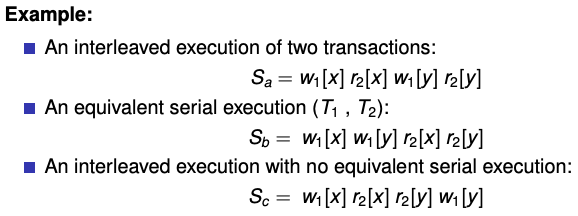recoverable schedule: no operations are non-recoverable

cascadeless schedule (ACA): does not have cascading abort

• if $$w_i[x], ..., r_j[x]$$ then must also have $$c_i, ..., c_j$$
• every cascadeless schedule is also recoverable

For every operation that a scheduler receives from a query processor, it can:

• execute it: send to lower module
• delay it: insert to queue
• reject it: cause abort of transaction
• ignore it: no effect

conservative scheduler: favours delaying operations

aggressive scheduler: favours rejecting operations

### Two Phase Locking (2PL)

Transactions must have a lock on objects before access

• shared lock for reading an object
• exclusive lock for writing an object

2PL protocol: transaction has to acquire all locks before it releases any of them

Theorem: 2PL guarantees the produced transaction schedules are (conflict) serializable

STRICT 2PL: locks held until commit, avoids cascading aborts (ACA)

### Isolation Levels in SQL

4 isolation levels:

• level 3 (serializability): table-level strict 2PL
• level 2 (repeatable read): tuple-level strict 2PL
• phantom tuple may occur (results from insert/delete during “all records” query)
• level 1 (cursor stability): tuple-level exclusive-lock only strict 2PL
• reading same object twice may result in different values
• level 0: neither read nor write locks are required, transaction may read uncommitted updates

### Recovery

Goals:

• allow transactions to be
• committed: with a guarantee that the effects are permanent, or
• aborted: with a guarantee that the effects disappear
• allow the database to be recovered to a consistent state in case of HW failure

Approaches to recovery:

• copy-on-write and merge-on-commit
• poor clustering
• not used in modern systems
2. logging
• use of log (separate disk) to avoid forced writes
• utilization of buffers
• preserves original clusters

#### Log Based Approaches

Logs are read/append only, and transactions add log records about what they do.

Types of information:

• UNDO: old versions of modified objects, used to undo changes made by a transaction which aborts
• REDO: new versions of modified objects, used to redo changes made by a transaction which commits
• BEGIN/COMMIT/ABORT: recorded whenever a transaction changes into one of these states

Log example: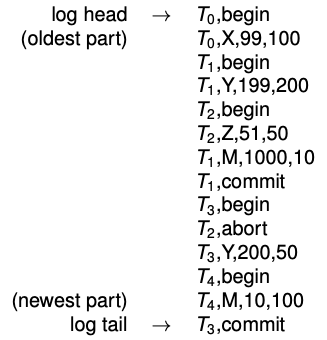To ensure the log is consistent with main database, use write ahead log (WAL). Requires:

• UNDO rule: a log record for an update is written to log disk before the corresponding data is written to the main disk
• guarantees atomicity
• REDO rule: all log records for a transaction are written to log disk before commit
• guarantees durability

## Database Tuning

physical design: process of selecting physical schema (data structures) to implement a conceptual schema

tuning: periodically adjusting physical or conceptual schema to adapt to requirements via:

• making queries run faster
• making updates run faster
• minimizing congestion due to concurrency

Both of the above rely on understanding the workload.

• most important queries/updates and their frequency
• desired performance goal for each query/update
• include the relations/attributes accessed/retrieved, selectiveness of join conditions

### Physical Schema

Storage strategy for each relation. Possible options:

• unsorted (heap) file
• sorted file
• hash file

• speed up queries
• increase update overhead

#### Indexes

Create/drop syntax:

CREATE INDEX LastnameIndex
ON Employee(Lastname) [CLUSTER]

DROP INDEX LastnameIndex


Effects of index:

• reduce execution time for selections with conditions involving Lastname
• increase insertion execution time
• increase or decrease execution time for updates or deletions of rows
• increase amount of space required to represent Employee

An index on attribute $$A$$ of a relation is clustering if tuples with similar values for $$A$$ are stored together in the same block.

Other indices are non-clustering.

A relation has at most one clustering index.

Two relations are co-clustered if their tuples are interleaved within the same file.

• useful for hierarchical data (1:n relationship)
• can speed up joins (particularly foreign key join)
• sequential scans of either relation slow down

Creating multi-attribute index:

CREATE INDEX NameIndex
ON Employee(Lastname, Firstname)

• tuples are organized first by Lastname, then Firstname
• useful for:
SELECT *
FROM Employee
WHERE Lastname = 'Smith'

SELECT *
FROM Employee
WHERE Lastname = 'Smith'
AND Firstname = 'John'

• extremely useful for:
SELECT Firstname
FROM Employee
WHERE Lastname = 'Smith'

SELECT Firstname, Lastname
FROM Employee

• not useful at all for
SELECT *
FROM Employee
WHERE Firstname = 'John'


### Tuning the Conceptual Schema

Unlike physical schema changes, conceptual schema changes often can’t be completely masked from end users.

normalization: process of decomposing schemas to reduce redundancy

denormalization: process of merging schemas to intentionally increase redundancy

Generally redundancy increases update overhead due to change anomalies, but decreases query overhead (due to less joins?).

partitioning: of a table means to split it into multiple tables to reduce bottlenecks

• horizontal: each partition has all the original columns and a subset of original rows
• separates operational from archival data
• vertical: each partition has a subset of original columns and all the original rows
• separates frequently used columns from eachother or from infrequently used columns

### Tuning Queries

Physical and conceptual schema changes impact all queries.

Guidelines:

• sorting (ORDER BY, DISTINCT, GROUP BY) is expensive
• when possible, replace subqueries with joins
• when possible, replace correlated subqueries with uncorrelated subqueries
• use vendor tools to examine query execution plan

### Tuning Applications

Guidelines:

• minimize communication costs
• return fewest columns and rows necessary
• update multiple rows with WHERE rather than a cursor
• minimize lock contention and hot spots
• delay updates and operations on hot spots as long as possible
• shorten/split transactions as much as possible
• perform insert/update/delete in batches
• consider lower isolation levels